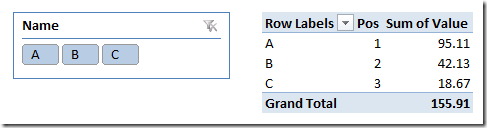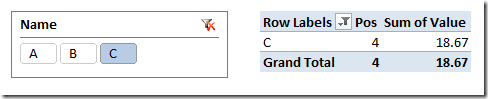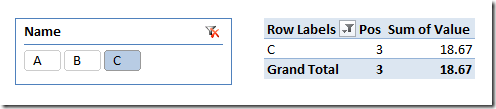Using decimal numbers in Power Pivot and Tabular might produce small rounding differences in certain calculations. This is nothing new when you work with floating point, as many programmer knows. The implementation of RANKX might suffer of a behavior producing wrong results when the measures used for the ranking returns a decimal value.

For example, consider the following model, where there are three names (A, B, C), each one with a value resulting from the sum of rows in the fact table and a Pos measure, calculated using the following measure:

Pos :=
IF (
HASONEVALUE ( Sample[Name] ),
RANKX (
ALL ( Sample[Name] ),
CALCULATE ( SUM ( Sample[Value] ) )
)
)In this case, everything works fine and the Pos has values from 1 to 3. However, when you select only one name, you might see a wrong number. In the following example, the Pos value is higher than the number of available names.It is not easy to find a reproducible case, usually the rounding error results from complex calculations. The purpose of the previous example is to describe the symptoms that you might experience.

Under the cover, the RANKX calculate the value of the measure for each element of the list of names, and then it searches in that table the result of the expression for the current filter context. If there is any rounding error in this operation… the match does not happen (or it might happen with the wrong index, even if this is harder) and you see the wrong Pos number as a result.

Hopefully, a fix to this behavior will be released sooner or later. In the meantime, there are two possible workarounds:

1. Cast the expression to currency using the CURRENCY function, so that the values compared are of currency data type, which is not subject to the described issue
2. Store the original value in a column of Currency data type, so that the result is still a currency and the match works well

By using either one of the workarounds, you will see the correct result:The first approach (cast the result) might have a minimal impact in query performance. I would prefer, whenever possible, storing the values in a Currency column, so that any measure will not suffer of this issue.

In any case, be careful about the data type of the expressions using in a RANKX function.

RANKXReturns the rank of an expression evaluated in the current context in the list of values for the expression evaluated for each row in the specified table.

`RANKX ( <Table>, <Expression> [, <Value>] [, <Order>] [, <Ties>] )`

IFChecks whether a condition is met, and returns one value if TRUE, and another value if FALSE.

`IF ( <LogicalTest>, <ResultIfTrue> [, <ResultIfFalse>] )`

HASONEVALUEReturns true when there’s only one value in the specified column.

`HASONEVALUE ( <ColumnName> )`

ALLCALCULATE modifier

Returns all the rows in a table, or all the values in a column, ignoring any filters that might have been applied.

`ALL ( [<TableNameOrColumnName>] [, <ColumnName> [, <ColumnName> [, … ] ] ] )`

CALCULATEContext transition

Evaluates an expression in a context modified by filters.

`CALCULATE ( <Expression> [, <Filter> [, <Filter> [, … ] ] ] )`

SUMAdds all the numbers in a column.

`SUM ( <ColumnName> )`

CURRENCYReturns the value as a currency data type.

`CURRENCY ( <Value> )`